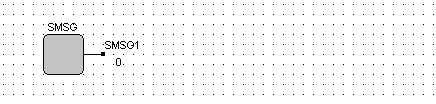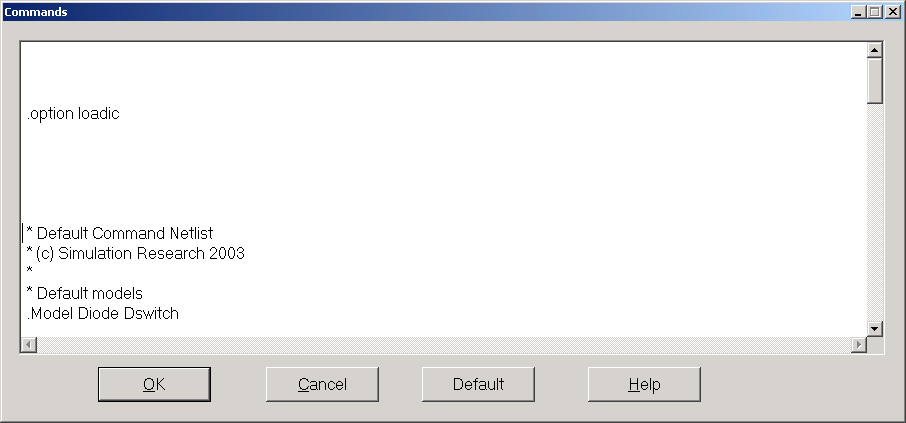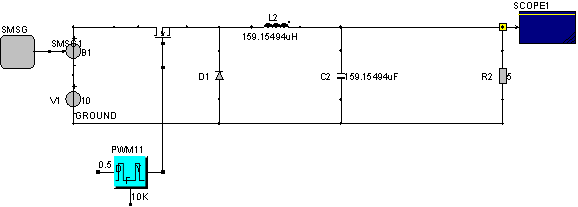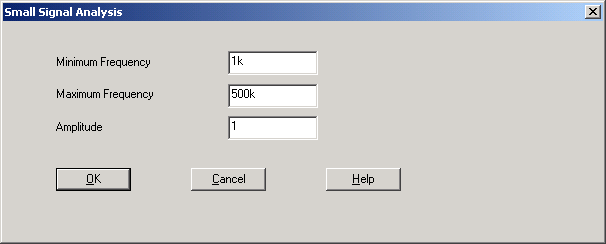• Introduction
• What is in this manual
• What is Caspoc
• User interface
• Introduction
• Starting
• Simulation
• Editing
• Viewing and printing
• Getting Started
• Basic editing
• Simulation in the time domain
• Basic User Interface Topics
• Editing
• Simulation
• Viewing
• Library
• Reports
• Project management
• Circuit and Block Diagram Components
• Introduction
• Cscript and user defined functions
• Component parameters
• Modeling Topics
• Introduction
• Power Electronics
• Semiconductors
• Electrical Machines
• Electrical drives
• Power Systems
• Mechanical Systems
• Thermal Systems
• Magnetic Circuits
• Green Energy
• Coupling to FEM
• Experimenter
• Analog hardware description language
• Embedded C code Export
• Coupling to Spice
• Small Signal Analysis
• Matlab coupling
• Tips and tricks
• Appendices

## Small Signal Analysis.

A small signal analysis is a synonym for determining an approximated linear model of a (non-) linear system. The small signal analysis in Caspoc determines a linear model by approximating a linear response during a simulation. Therefore the method is applicable to any type of converter, but with the restriction that a linear model can in theory be derived and exists.

When performing a small signal analysis, there are two things we have to keep in mind. First the small signal analysis is a linear approximation of the steady state. Therefore before the small signal analysis is started, the steady state of the converter has to be determined by a simulation. Secondly any perturbation disturbing the linear behavior will lead to erroneous results.

Performing the linearization on a switched mode power supply means the averaging of the voltages and currents over the switching intervals.

To perform a small signal analysis of a power converter you need the block "smsg " for the perturbation. This block can be added to a control signal or placed in series with a voltage source using a controlled voltage source "B". You can insert as many smsg block as you want. In each scope you can calculate the small-signal transfer function relative to the input smsg block.In older versions of Caspoc, in the commands dialog box the .option loadic has to be specified. Open the commands dialog by selecting Insert/Edit Commands from the menu. Add the following option:

.option loadicThe basic steps for perform the small signal analysis are outlined below:

1. Set the parameters for the analysis:
2. Define the frequency range, Fmin and Fmax.

Set Tscreen equal to 1/Fmin

Set the Amplitude of the perturbation to an appropriate value in the dialog box small-signal parameters, for example, 1 if the input voltage is 10 volt. (Remember that is a perturbation)

3. Simulate the circuit until steady state.
4. Save the initial conditions in the *.ic file.
5. Start the small signal analyses by selecting the small signal start button or selecting: "Simulation/Start small signal analysis"
6. The simulation will run two times. First for the steady state and secondly for the perturbation.
7. Open the scope with the right mouse button.
8. The small signal calculation is performed automatically.
9. The number of harmonics calculated is equal to: Scale/DFT Parameters : [Number of harmonics] in the scope and is not adjusted automatically.
10. Set the number of harmonics, for example 100 or 1000 and select: View/DFT. The small-signal transfer function is recalculated and displayed
11. Each time a new small-signal analysis is carried out where one of the circuit parameters is changed, proceed with step 2

In the next example we will perform a small signal analysis of a buck converter. Build the circuit as indicated below:The buck converter has a fixed frequency of 10kHz and fixed dutycycle of 50%. Although this circuit can be analyzed easily with the state space averaging, in this example the Bode Diagram of the input-output transfer function is calculated beyond the switching frequency. This shows that the method is independent on any switching frequency and dutycycle. The resulting Bode Diagram will show the filter characteristics for a second order filter.

First simulate the converter until the steady state is reached. Use Tscreen=1ms and dt=1us for the simulation. After reaching the steady state, save the initial condition for this steady state by selecting Tools/Save Initial Condition and save it under the same name as the *.csi file, but with the .ic extension.

The parameters for the small signal analysis have to be set in the dialog box "Small signal Parameters", which can be found in the menu simulation.

The minimum frequency is also dependen on Tscreen. Tscreen has to be at least 1/(Minimum Frequency). The maximum frequency is also limited by the integration step size used in the simulation. In theory the simulation is limited to half the sample frequency so the upper frequency limit is in this case 500kHz. The amplitude of the perturbation is set to 1, which means that the input voltage of 10 volts is perturbed by 10%.Start the small signala analysis by selecting the menu item "Simulation/Start Small Signal Analysis"The simulation will start and when finished you can open the scopes by clicking it with the right mouse button. The scope will open and first an analysis is performed. You will see a progress bar in the status bar of the scope.The output is displayed on a linear frequency scale or on a logarithmic frequency scale. You can set this by selecting in the scope "Scale/Edit left Scale" and mark the checkbox "Log. Freq. Scale". The amplitude is displayed in dB

Note!

Using the cursor keys the value of the amplitude and phase can be read. The cross is however not displayed in the correct position for the logarithmic representation.

The upper frequency limit for displaying the Bode diagram is specified at "Scale/DFT Parameters" Specify the number of harmonics to be displayed. After changing the number of harmonics, you have to select the menu item "View/DFT" to recalculate the transfer function and redisplay all harmonics.

You can have up to 20 traces/inputs in the scope. Both the magnitude and phase have the same color as the connection of the scope and the same color as the trace during the time domain simulation and multiple simulation.